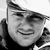# App Development

Discussion board where members can learn more about Qlik Sense App Development and Usage.

Announcements
Welcome to Qlik Community! Check out our new navigation! FIND OUT MORE
cancel
Showing results for
Did you mean:Partner - Contributor III

## Ingoring multiple filters in set analysis

Hi all

assume you have 10 filters (A, B, C ...) on a dashboard and a KPI that should apply only the first filter (A). All other filters have to be ignored in the calculation. Is there any syntax in set analysis to say "take only this filter ... an ignore all others"?

Thanks a lot

Labels (2)

• ### Set Analysis

1 Solution

Accepted SolutionsMVP

It should not ignore A.

=sum({1<[A]= P([A])>}Amount)

Try using the expression  on this datamodel.

``````Transactions:
TransLineID,
TransID,
Pick(Ceil(3*Rand1),'A','B','C') as D,
Pick(Ceil(6*Rand1),'a','b','c','d','e','f') as C,
Pick(Ceil(3*Rand()),'X','Y','Z') as B,
mod(TransID,26)+1 as A,
Round(1000*Rand()*Rand()*Rand1) as Amount;
Rand() as Rand1,
IterNo() as TransLineID,
RecNo() as TransID
Autogenerate 1000
While Rand()<=0.5 or IterNo()=1;``````

4 RepliesMVP

You could do something like this:

=sum({1<[A]= P([A])>}Amount)Partner - Contributor III
Author

no, sorry - in this case also A is ignoredMVP

It should not ignore A.

=sum({1<[A]= P([A])>}Amount)

Try using the expression  on this datamodel.

``````Transactions:
TransLineID,
TransID,
Pick(Ceil(3*Rand1),'A','B','C') as D,
Pick(Ceil(6*Rand1),'a','b','c','d','e','f') as C,
Pick(Ceil(3*Rand()),'X','Y','Z') as B,
mod(TransID,26)+1 as A,
Round(1000*Rand()*Rand()*Rand1) as Amount;
Rand() as Rand1,
IterNo() as TransLineID,
RecNo() as TransID
Autogenerate 1000
While Rand()<=0.5 or IterNo()=1;``````Partner - Contributor III
Author

you are right, I also tried it with another simple model and it works as you describe.

I will check, why it does not work in the other modell, but thanks a lot for your help.

Kind regards, AndyTags
Community Browser